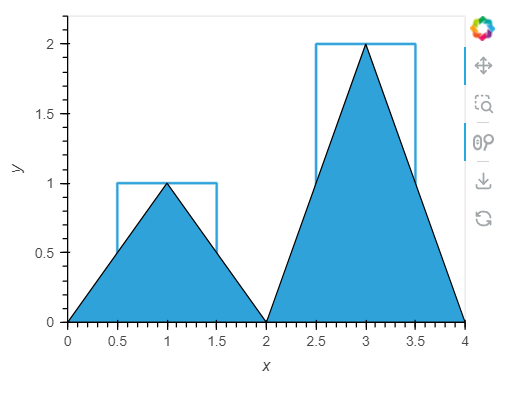# Hvplot step area plot feature request

An hvplot area plot that is composed of steps instead of lines would be an incredible feature.

It would make a plot like the following much more aesthetic and also much more accurate and practical.

Any thoughts?

1 Like

I believe it could be achieved with pandas `reindex` and `ffill` method, but I haven’t tested yet.

According to chatgpt it’s achievable by using holoviews. Would be nice to have directly in the hvplot api.

``````import numpy as np
import pandas as pd
import holoviews as hv

# Sample data
df = pd.DataFrame({
'x': [0, 1, 2, 3, 4],
'y': [0, 1, 0, 2, 0]
})

# Create a Curve with step interpolation
curve = hv.Curve(df, 'x', 'y').opts(interpolation='steps-mid')

# Create an Area plot under the curve
area = hv.Area(df, 'x', 'y')

# Combine the curve and area
step_area = (curve * area).opts(width=400, height=300)

step_area

``````
1 Like

How would ChatGPT explain that it looks like below?``````import numpy as np
import pandas as pd
import holoviews as hv
import panel as pn

pn.extension()
hv.extension("bokeh")

# Sample data
df = pd.DataFrame({
'x': [0, 1, 2, 3, 4],
'y': [0, 1, 0, 2, 0]
})

# Create a Curve with step interpolation
curve = hv.Curve(df, 'x', 'y').opts(interpolation='steps-mid')

# Create an Area plot under the curve
area = hv.Area(df, 'x', 'y')

# Combine the curve and area
step_area = (curve * area).opts(width=400, height=300)

pn.panel(step_area).servable()
``````
1 Like